computer programsJOURNAL OFSYNCHROTRONRADIATION
ISSN: 1600-5775
Volume 20| Part 1| January 2013| Pages 200-204

DetOx: a program for determining anomalous scattering factors of mixed-oxidation-state species

aChemical Crystallography, Chemistry Research Laboratory, Mansfield Road, University of Oxford, Oxford OX1 3TA, UK, and bDiamond Light Source, Didcot, Oxfordshire, UK
*Correspondence e-mail: richard.cooper@chem.ox.ac.uk

(Received 19 July 2012; accepted 23 October 2012; online 13 November 2012)

Overlapping absorption edges will occur when an element is present in multiple oxidation states within a material. DetOx is a program for partitioning overlapping X-ray absorption spectra into contributions from individual atomic species and computing the dependence of the anomalous scattering factors on X-ray energy. It is demonstrated how these results can be used in combination with X-ray diffraction data to determine the oxidation state of ions at specific sites in a mixed-valance material, GaCl2.

1. Introduction

Anomalous scattering (AS) is an interaction that occurs when the energy of an incident X-ray photon is close to an electronic resonance of a bound inner electron. The result is a change in phase of the diffracted X-ray beam, which can be exploited to provide phasing information for techniques such as multiple-wavelength anomalous diffraction (Hendrickson & Ogata, 1997; Hendrickson et al., 1985; Hendrickson, 1991). To account for the phase change, the normal atomic (Thompson) scattering factor, f0, is modified such that f = f0 + f ′ + if ′′. The absorption of X-rays at resonant energies (the absorption edge) can be characterized by measuring the absorption or fluorescence with respect to the incident photon energy. From this we are able to compute the real, f ′, and imaginary, f ′′, parts of the AS factors at energies on and around an absorption edge.

1.1. Theory versus experiment

Experiments to determine the oxidation state of an atom within a single crystal can be carried out given precise knowledge of the atomic scattering factors close to an absorption edge (Wilkinson et al., 1991). Fluorescence-absorption experiments are routinely applied in macromolecular crystallography to find the absorption-edge positions of heavy atoms, e.g. Se, that have been substituted into the crystals (Terwilliger, 1994). Theoretical values for the real and imaginary parts of the anomalous scattering factor can be computed using two equations. The first equation, the optical theorem (James, 1969), directly relates the absorption-fluorescence data to the imaginary, f ′′, part of the AS factor,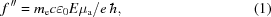where me is the mass of an electron, 0 is the permittivity of free space constant, E is the incident X-ray energy, and μa is the atomic absorption coefficient. The real part of the AS factor is determined via a numerical integration of the Kramers–Kronig transformation,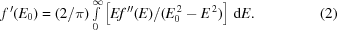These calculations, however, only apply to isolated atoms and, therefore, deviations from calculated values are observed in experimental data. These deviations are most noticeable in the vicinity of the absorption edge (X-ray absorption near-edge structure, XANES, and extended X-ray absorption fine structure, EXAFS), caused by the chemical environment around the absorbing species interfering with ejected photoelectrons (Koningsberger & Prins, 1988; Fig. 1). Calculation of the real and imaginary components of the AS from the fluorescence experiment is implemented in the program CHOOCH (Evans & Pettifer, 2001) for cases where there are no overlapping absorption edges.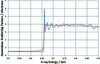Figure 1 The effect of EXAFS and XANES on imaginary AS factors. The dotted red line shows the theoretical values for a Zn(0) species and the solid blue line shows the calculated spectrum from a sample of octaethyl-porphine zinc.

1.2. Anomalous scattering of mixed-valence materials

Since the position of the absorption edge is dependent upon electronic transitions within an atom, increasing the oxidation state results in the absorption edge moving to higher energy. As the number of valence electrons is decreased, the shielding of the nuclear charge from the core 1s electrons is also decreased, which in turn increases the K-shell absorption energy required to eject one of the core electrons. Measurements on a sample of GaCl3, containing only Ga(III) and Cl atoms, give an absorption edge at 10375 eV, cf. 10367 eV for the theoretical Ga(0) atom (Cromer & Lieberman, 1970, 1981; Cromer, 1983). The dependence upon absorption-edge position as a function of oxidation state means that the fluorescence spectrum for a species containing two or more oxidation states of the same element is effectively a sum of the spectra from the individual atoms in their different oxidation states and atomic environments. The rapid variation of absorption with energy approaching the absorption edge means that at certain energies the AS factors will be significantly different for each oxidation state.

2. DetOx

DetOx is a program, written using Python (Rossum, 1995), which deconvolutes the absorption-fluorescence spectrum of a mixed-oxidation state compound into its component spectra for each atomic oxidation state present in the sample. Input into DetOx is in the form of a plain text file containing two columns (space or tab delimited): X-ray energy in electronvolts and corresponding intensity-corrected absorption-fluorescence values on an arbitrary scale. The program also requires input of the element under inspection and the number of expected oxidation states of the atom. There is an additional option to manually input absorption peak energies if the program is unable to detect them.

2.1. Normalization

Data are corrected for incident beam intensity before being normalized. This is performed by dividing the fluorescence signal at a given energy by the equivalent absorption reading from a diode placed in the beam, with the sample removed. Fluorescence data are input on an arbitrary scale, since there are many factors other than just the nature of the atomic scatterer that can contribute to the signal. These include the energy of the incident X-ray beam, volume of the sample, the geometric orientation of the crystal on the diffractometer, the partial concentrations of different oxidation state heavy-atoms in the sample and the distance of the sample from the detector. Background from elastically scattered X-rays and other sources also becomes an issue when making accurate measurements (Evans & Pettifer, 2001). Therefore, the recorded fluorescence data must undergo a background correction and normalization. The input spectrum is normalized such that below the edge the signal tends to zero and above the edge the signal tends to unity, leaving only features caused by EXAFS and XANES around the absorption edge.

The normalization is achieved by fitting a straight line to input fluorescence data (Fraw) more than 50 eV below the theoretical edge (Φbelow) and a second to data more than 50 eV above the edge (Φabove). These lines are extrapolated across the whole range of the data and then used to adjust the raw data to leave the normalized spectrum [N(E)],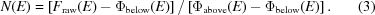In order for the program to normalize the data accurately, data should ideally be collected to a range ±200 eV from the theoretical absorption edge, to give reliable extrapolation of Φbelow and Φabove. Away from the absorption edge (±200 eV), EXAFS and XANES have a negligible effect on the fluorescence signal so it can be assumed that calculated theoretical values are an accurate approximation for the normalized spectrum (Evans & Pettifer, 2001).

2.2. Determination of f ′′

Theoretical values of f ′′ over the energy range of the experimental data are obtained using the program MuCal (Bandyopadhyay & Segre, undated). The values above and below the edge can be described with Victoreen polynomials; however, over this narrow energy range linear functions (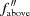and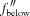) are sufficient to give an accurate fit. The experimental f ′′ spectrum is therefore calculated by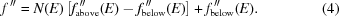2.3. Deconvolution

As demonstrated by Wilkinson & Cheetham (1992), the GaCl2 spectrum is a sum of the fluorescence curves for the Ga(I) and Ga(III) species in the sample. Since it is not possible to determine exactly how the EXAFS above the edge differ between the two oxidation states, we have made the assumption that the post-absorption-edge EXAFS features do not have an effect on the position of the f ′ peak. We have tested this assumption by replacing the data points after the absorption edge with theoretical values and determining the related f ′ values. As shown in Fig. 2, despite the post-edge EXAFS features being removed from the f ′′ spectrum, the position of the f ′ peak remains unchanged. Since we are only interested in the features of spectra before the absorption-edge peak, it is not critical to determine the exact contributions to the EXAFS region from individual oxidation state species, as this will not have a significant effect on the AS factors.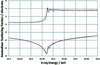Figure 2 The effect of EXAFS on the peak position in the real scattering factors. The dotted blue line shows the DetOx output for the GaCl2 data assuming only one oxidation state is present and the solid red line shows the deconvoluted Ga(I) oxidation state data (with EXAFS removed).

To overcome the problem of overlapping signals in the spectrum, a Fourier deconvolution is utilized. The measured spectrum, y(E), is defined as a convolution of the ideal signal, s(E), and a response function, r(E), plus a uniform noise function, n(E). If we assume that the signal itself is the sum of two identical underlying fluorescence curves, u(E) and u(E + ΔE), where one is offset by a shift of ΔE, then the signal, s(E), may be expressed as a convolution u(E)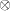d(ΔE), where d(E) is the sum of two appropriately scaled δ functions separated by ΔE, m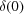+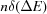. The peaks in the d(E) function are scaled such that the ratio of the areas under each, m:n, is proportional to the ratio of the oxidation states present in the sample. In the case of GaCl2, this ratio is simply 1:1,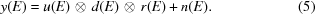In order to determine AS coefficients for each species we must determine the shape of the function u(E). This may be achieved using a Fourier deconvolution. In the absence of noise, we could recover our unknown data signal by taking the reverse Fourier transform of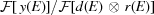. The optimal solution in the presence of noise, n(E), is found by applying a Wiener filter (Press et al., 2007) to this expression, the effect of which is to attenuate the influence of terms with a low signal-to-noise ratio in the denominator.

In cases where only one oxidation state exists in the molecule, a single Gaussian can be used for the response function in the above equation. The effect in this case is simply to remove some broadening of the signal caused by X-ray monochromator bandpass, detector precision and other random effects. Using an iterative process, we found that the optimum width for the response function, which sharpened the signal without introducing unexpected pre-edge features, was 0.16 eV. This value is expected to be instrument-specific. For mixed-oxidation-state cases, we employ a second iterative process to determine the optimum value of ΔE subject to the conditions that the original signal is reproduced by application of (3)and no unexpected features are introduced to the extracted absorption edge, u(E). The output consists of two f ′′ curves, separated by a value of ΔE eV, that when added together reproduce the original input absorption-fluorescence signal. These curves are normalized to theoretical data away from the edge to give the imaginary AS factors for the different oxidation states as described in §2.

2.4. Determination of f ′

Firstly a Savitzky–Golay filter (Gorry, 1990) is applied to the deconvoluted curves in order to smooth the data. The f ′ values are then calculated by performing the Kramers–Kronig transformation, integrating between 0 andat each energy, (E0), in the f ′′ spectrum. However, the denominator of the integration (E02E2) contains a singularity, over which it is not possible to integrate. This can be overcome numerically after a Taylor expansion of the integral as described by Hoyt et al. (1984). The integration is performed using the Python Quadrature module, from the Numpy and SciPy open-source libraries (Jones et al., 2001), which computes a definite integral using fixed-tolerance Gaussian quadrature. As implemented, the integration is performed between suggested limits of 0 eV and 50 times the theoretical edge energy (Hoyt et al., 1984), to ensure the program runs quickly. Owing to the assumption made in the deconvolution that both underlying signals are the same shape, the values of f ′ will be most reliable below the absorption edge, where there are no significant differences between the shapes of the two curves.

2.5. DetOx output

The default output from DetOx is a graph showing both f ′′ and f ′ calculated from the deconvoluted signals. The program will also tabulate the AS factors for all oxidation states present in the sample at given energies and output, a graph of the normalized spectrum, the effective f ′′ spectrum before the deconvolution and the data for the deconvoluted spectrum in plain text format.

3. Experimental

Fluorescence-absorption data and X-ray diffraction data were all collected on the Small-Molecule Single-Crystal Diffraction beamline, I19, at Diamond Light Source. Full diffraction datasets were collected in EH1 on a custom-built Rigaku CrystalLogic diffractometer, using a Saturn724+ CCD detector at 150 K (Nowell et al., 2012). A silicon double-crystal monochromator is used on the beamline for selecting a wavelength by changing its incident angle with the X-ray beam.

Fluorescence-absorption data were collected using a Vortex detector mounted next to the sample. As the monochromator angle and hence the wavelength is driven through a chosen range at set intervals, the Vortex detector records the X-ray fluorescence emitted by the sample. Initially, a broad scan (∼5 eV intervals) was recorded to locate the position of the absorption edge. A much finer scan was then recorded (∼0.25 eV intervals) across the absorption edge (±40 eV) in order to see the exact edge position and near-edge features.

The background-corrected data were then input into DetOx. Using the program output, appropriate wavelengths were chosen for data collection so as to maximize the differences in f ′′ and f ′ between two oxidation states. DetOx provided the calculated AS factors at the chosen wavelengths. Once the structure has been solved, the relevant AS factors were input into CRYSTALS (Betteridge et al., 2003) to determine the oxidation state of an element at a given site.

GaCl3, containing just one oxidation state, Ga(III), was used as a test sample and results of the normalization and Kramers–Kronig transformation from DetOx were verified against those from CHOOCH (Evans & Pettifer, 2001; Fig. 3).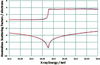Figure 3 Comparisons between the output from DetOx (dotted blue line) and CHOOCH (solid red line) for a sample of GaCl3.

3.1. GaCl2: a mixed-oxidation-state compound

GaCl2 was chosen for this study since it has been extensively studied and the structure has been reported (Garton & Powell, 1957; Schmidbaur et al., 1987; Wilkinson et al., 1991). A similar experiment to exploit the anomalous signal in order to determine the oxidation state at each Ga position within the structure using powder diffraction data has been previously reported by Wilkinson & Cheetham (1992).

Single crystals were obtained from Sigma Aldrich in a sealed vial under nitrogen. Crystals were immersed in dried oil under nitrogen and mounted directly in the cold stream to reduce exposure time to air and moisture.

The theoretical absorption edge of Ga is 10367 eV. Therefore, absorption-fluorescence data were collected between 10100 eV and 10700 eV in steps of 5 eV to locate the absorption edge. After an initial analysis of the output from DetOx, a finer scan was performed between 10345 eV and 10415 eV in steps of 0.25 eV. Full X-ray diffraction data collections were then recorded at 10314 eV (below the absorption edge) and 10368 eV [on the Ga(I) absorption edge]. Using CRYSTALS it was possible to determine the oxidation states of the Ga atoms in the structure. Firstly, each Ga site was occupied by two atomic species, Ga(I) and Ga(III), with AS factors calculated in DetOx (Table 1), and with occupancies summing to unity. The expectation would be that the occupancy of the atom with the Ga(I) AS factors refines to unity at the Ga(I) site and zero at the Ga(III) site and vice versa for the Ga atom with the Ga(III) AS factors.

 Table 1Anomalous scattering factors output from DetOx
Dataset Oxidation state f ′′ f ′
On edge Ga(I) 2.41 −7.36
10368 eV Ga(III) 1.88 −7.00
Below edge Ga(I) 0.59 −4.69
10314 eV Ga(III) 0.59 −4.62

4. Results and discussion

The output from DetOx is shown in Fig. 4(a), and Fig. 4(b)shows the deconvoluted f ′′ superimposed onto the normalized fluorescence spectrum of GaCl2 before deconvolution. Table 1shows the anomalous scattering factors, calculated by DetOx.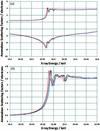Figure 4 (a) Output from DetOx from a sample of GaCl2. The solid red lines show the AS factors for the Ga(I) ions and those for the Ga(III) are shown by the dotted blue line. (b) Overlay of the two deconvoluted curves {solid red [Ga(I)] and blue [Ga(III)] lines} superimposed onto the original spectrum for GaCl2 (dotted black line).

Structures were solved for both datasets using SIR97 (Altomare et al., 1999) and all refinement and analysis was undertaken using the CRYSTALS crystallographic package. The coordinates for atoms in the crystal structure were consistent with previous single-crystal studies by Schmidbaur et al. (1987). A filter of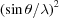< 0.145 (based on examination of the Wilson plot) was added to remove the weak high-angle data. The results from the occupancy refinement are shown in Table 2.

 Table 2Refined occupancies from CRYSTALS
Dataset Ga Site Ga(I) Ga(III)
On edge Ga(I) 1.02 (20) −0.02 (20)
10368 eV Ga(III) −0.02 (20) 1.02 (20)
Below edge Ga(I) 1.3 (15) −0.3 (15)
10314 eV Ga(III) −0.3 (15) 1.3 (15)

The DetOx output revealed an optimum energy offset between the Ga(I) and Ga(III) absorption edges, consistent with that reported by Wilkinson & Cheetham (1992) from powder data, of 3.3 eV. The values in Table 2show that the occupancies refine as expected; however, the reliability of these values must be taken into consideration. Since the errors in occupancy for the on-edge dataset (±0.20) are much lower than those below the edge (±1.5), it is clear that the values from the below-edge analysis are less reliable. The reason for this is that the below-edge AS values are identical to within ±0.05 eV (much less than the electron density of an H atom), which results in a strong correlation between the Ga(I) and Ga(III) occupancies. However, on the Ga(I) absorption edge, where f ′′ varies rapidly with energy, the difference is much larger allowing the occupancies to be determined more reliably.

The accuracy of the f ′ data has been verified by the test spectrum shown in Fig. 3on GaCl3. Therefore, the main source of potential error arises in the deconvolution step. Fig. 4(b)shows that the deconvoluted curves align with the appropriate peaks in the original spectrum. Even so, the assumption that the curves have the same form up to the absorption peak (the section of the f ′′ spectrum we are interested in) is difficult to test. Nevertheless, the results presented in Table 2support the accuracy of the calculated AS factors, and indicate that this assumption is appropriate for the function of the program.

5. Conclusion

DetOx can be used to discriminate between different oxidation states of the same element within a mixed-oxidation-state compound using tunable synchrotron radiation. By analysing the output from DetOx, an appropriate wavelength can be selected in order to maximize the differences in f ′ and f ′′. Values of the AS factors at the selected wavelengths can then be determined and input into an appropriate refinement program such as CRYSTALS. Occupancies can be refined at each site to determine the oxidation state of an atom. Results from single-crystal studies on GaCl2 are consistent with previous reports from powder data. DetOx will be published on-line and is currently available from the authors.

Acknowledgements

We acknowledge Diamond Light Source and The Department of Chemistry, University of Oxford, for funding KJS's studentship, Dr Gwyndaf Evans for helpful discussions about theoretical and practical aspects of the implementation, and Mark Irwin for assistance with sample preparation. We would also like to thank Diamond Light Source for the award of beam time (MT7768) to carry out the data collections, and the referees of this manuscript for their helpful comments and questions.

ReferencesJOURNAL OFSYNCHROTRONRADIATION